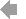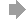Basic HTML VersionView Full Version
Page 221 - Course_Catalog_2020-2021
P. 221

``````All courses are for unit credit and apply to a De Anza associate degree unless otherwise noted.
M MATH 76 MATH 76X
Special Projects in Probability and Statistics
1 Unit
2 Units 3 Units
MATH 211X Algebra Support for Finite 2 1/2 Units Mathematics
Credit course - Does not apply to De Anza associate degree. Corequisite: MATH 11 or MATH 11H.
Two and one-half hours lecture (30 hours total per quarter). Pass-No Pass (P-NP) course.
A review of the core prerequisite skills, competencies, and concepts needed when studying linear functions, exponential and logarithmic functions, and probability and optimization models. Intended for students who are concurrently enrolled in Finite Mathematics
MATH 76Y
Prerequisite: Consent of instructor and division dean.
Three hours laboratory for each unit of credit (36 hours total for each unit of credit per quarter).
Pass-No Pass (P-NP) course.
Individual special reading, writing or study projects in probability and statistics as determined in consultation with the instructor.
MATH 212
College Math Preparation Level 2:
5 Units
MATH 77
MATH 77X
MATH 77Y
Prerequisite: Consent of instructor and division dean.
Three hours laboratory for each unit of credit (36 hours total for each unit of credit per quarter).
Pass-No Pass (P-NP) course.
Individual special reading, writing, or study projects in mathematics as determined in consultation with the instructor.
Special Projects in Mathematics
1 Unit 2 Units 3 Units
Beginning Algebra
MATH 78
MATH 78X
MATH 78Y
Prerequisite: Consent of instructor and division dean.
Three hours laboratory for each unit of credit (36 hours total for each unit of credit per quarter).
Pass-No Pass (P-NP) course.
Individual special reading, writing, or study projects in pure mathematics as determined in consultation with the instructor.
Credit course - Does not apply to De Anza associate degree.
Five hours lecture (60 hours total per quarter).
Application of linear functions, quadratic functions and linear systems to problems. Emphasis on the development of models of real-world applications and interpretation of their characteristics.
MATH 217 Integrated Statistics 1 10 Units
Credit course - Does not apply to De Anza associate degree.
Prerequisite: Qualifying score on the Math Placement Test within last calendar year; or MATH 210 or equivalent with a grade of C or better.
Ten hours lecture (120 hours total per quarter).
This is the first quarter of two in the Statway sequence comprised of MATH 217 and MATH 17. This sequence covers concepts and methods of statistics with an emphasis on data analysis. Topics include methods for collecting data, graphical and numerical descriptive statistics, correlation, simple linear regression, non-linear models and basic concepts of probability. The course introduces the student to applications in engineering, business, economics, medicine, education, the sciences, and those pertaining to issues of contemporary interest. Where appropriate, the contributions to the development of statistics by men and women from diverse cultures will be introduced. This sequence is recommended for students with majors that require no mathematics beyond freshman-level statistics. It is not appropriate for students with majors in math, science, computer science or business, nor for students desiring to transfer to a private university.
MATH 231 Algebra Support for Precalculus I 2 1/2 Units
Corequisite: MATH 31, 41, or 41H.
Two and one-half hours lecture (30 hours total per quarter).
Pass-No Pass (P-NP) course.
A review of the core prerequisite skills, competencies, and concepts needed in when studying polynomial, rational, exponential and logarithmic functions. Intended for majors in business, science, technology, engineering, and mathematics who are concurrently enrolled in Precalculus I.
MATH 231A Algebra Support for Precalculus I 2 1/2 Units (Part 1)
Corequisite: MATH 31A.
Two and one-half hours lecture (30 hours total per quarter).
Pass-No Pass (P-NP) course.
A review of the core prerequisite skills, competencies, and concepts needed when studying polynomial and rational functions. Intended for majors in business, science, technology, engineering, and mathematics who are concurrently enrolled in Precalculus I.
Special Projects in Pure Mathematics
1 Unit 2 Units 3 Units
Special Projects in Applied Mathematics
1 Unit 2 Units 3 Units
MATH 79
MATH 79X
MATH 79Y
Prerequisite: Consent of instructor and division dean.
Three hours laboratory for each unit of credit (36 hours total for each unit of credit per quarter).
Pass-No Pass (P-NP) course.
Individual special reading, writing, or study projects in applied mathematics as determined in consultation with the instructor.
MATH 109 Intermediate Algebra for Statistics 5 Units
Five hours lecture (60 hours total per quarter).
Applications of linear and exponential functions. Emphasis on the development of models of real world applications and interpretation of their characteristics. Introduction to discrete probability, and data analysis, making use of graphical and numerical techniques.
MATH 114 College Math Preparation Level 3: 5 Units Intermediate Algebra
Prerequisite: MATH 212 or equivalent placement.
Five hours lecture (60 hours total per quarter).
Application of exponential, logarithmic, and rational functions. Emphasis on the development of models of real world applications and interpretation of their characteristics.
MATH 130 Intermediate Algebra for Precalculus 5 Units
Five hours lecture (60 hours total per quarter).
Application of linear functions, quadratic functions, exponential functions, logarithmic functions and linear systems. Emphasis on the development of models of real-world applications and interpretation of their characteristics.
MATH 231B
Corequisite: MATH 31B.
Two and one-half hours lecture (30 hours total per quarter).
Pass-No Pass (P-NP) course.
A review of the core prerequisite skills, competencies, and concepts needed when studying exponential and logarithmic functions. Intended for majors in business, science, technology, engineering, and mathematics who are concurrently enrolled in Precalculus I.
MATH 232 Algebra Support for Precalculus II 2 1/2 Units
Corequisite: MATH 32, 42, or 42H.
Two and one-half hours lecture (30 hours total per quarter).
Pass-No Pass (P-NP) course.
A review of the core prerequisite skills, competencies, and concepts needed in studying the theory of trigonometric functions and their applications. Intended for majors in business, science, technology, engineering, and mathematics who are concurrently enrolled in Precalculus II.
MATH 241 Academic Excellence in Precalculus I 1 Unit
Credit course - Does not apply to De Anza associate degree. Corequisite: MATH 41 or MATH 41H.
Advisory: EWRT 211 and READ 211, or ESL 272 and 273. Three hours laboratory (36 hours total per quarter).
MATH 210 College Math Preparation Level 1: Pre-Algebra
5 Units
Credit course - Does not apply to De Anza associate degree.
Five hours lecture (60 hours total per quarter).
Use of basic arithmetic in application problems, estimation, the real number system, variables and linear equations, graphs of linear equations and the Cartesian coordinate system, the concept of function.
MATH 210X Support for Statistics
Credit course - Does not apply to De Anza associate degree. Corequisite: MATH 10 or MATH 10H.
Two and one-half hours lecture (30 hours total per quarter). Pass-No Pass (P-NP) course.
2 1/2 Units
A review of the core prerequisite skills, competencies, and concepts needed when studying probability and statistics. Intended for students who are concurrently enrolled in Statistics.
220
2O20-2O21 DE ANZA COLLEGE CATALOG
Algebra Support for Precalculus I
2 1/2 Units
(Part 2)
``````219   220   221   222   223